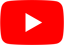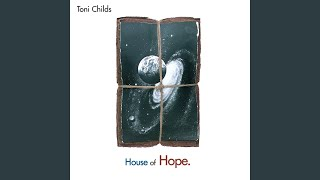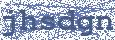Where's The Light
Toni Childs

## TONI CHILDS

Where's The Light Lyrics

And you're sound some peace
But I don't think the money's
Gonna take away the need

And yes I'm hurting girl
You won't see it much
'Cause it's the stuff we don't let out
It's what you will not touch

What it is now heart?
What we need to find?
It seems we're all lookin' for
Some peace of mind

Where's the light, where's the light
In the darkness of my life
Where's the light, where's the light
In the darkness there is no light

What you're lookin' for?
What you're lookin' for?

To be satisfied
To go underground
To swim inside the ocean
Descend to the profound

To reach the bedrock
A quest, a psychic death
A wild thought, I just caught
Would this be the end?

Can you help me now?
How to find
Some peace and relief?
Where's the light, where's the light
In the darkness of my life
Where's the light, where's the light
In the darkness there is no light

What you're lookin' for?
What you're lookin' for?
What you're lookin' for?

Where's the light, where's the light
In the darkness of my life
Where's the light, where's the light
In the darkness there is no light
There is no light

Where's the light, where's the light
In the darkness of my life
Where's the light, where's the light
In the darkness there is no light

Where's the light, where's the light
In the darkness of my life
Where's the light, where's the light
In the darkness there is no light

Where's the light, where's the light
In the darkness of my life
Where's the light, where's the light
In the darkness there is no lightHottest Lyrics with Videos
02eb83fcf843ce4fb2b86c63b9e09671